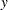COORDINATES Statement
COORDINATES coordinate-variables ;

The two options in the COORDINATES statement give the name of the variables in the DATA= data set that contains the values of theandcoordinates of the conditioning data. You must specify the COORDINATES statement when you specify an input data set with the DATA= option in the PROC SIM2D statement.

Only one COORDINATES statement is allowed, and it is applied to all SIMULATE statements that have the VAR= specification. In other words, it is assumed that all the VAR= variables in all SIMULATE statements have the sameandcoordinates.

You can abbreviate the COORDINATES statement as COORD.

XCOORD=(variable-name)
XC=(variable-name)

gives the name of the variable that contains thecoordinate of the data in the DATA= data set.

YCOORD=(variable-name)
YC=(variable-name)

gives the name of the variable that contains thecoordinate of the data locations in the DATA= data set.